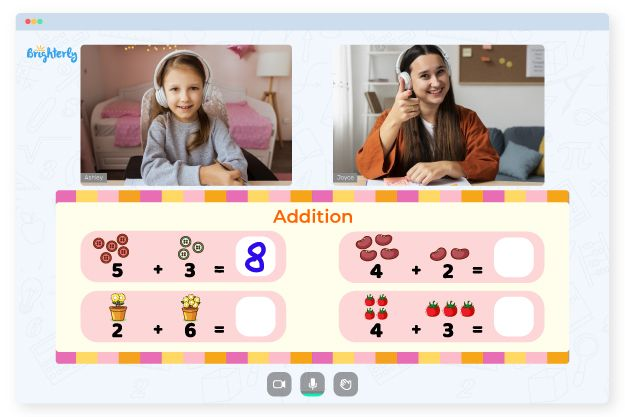# 2 Digit Addition With Regrouping Worksheets

If you are a teacher or parent of a student in second grade or higher, your kid must have started learning how to add two-digit numbers. But depending on the way you approach the topic, this task may quickly become too challenging. Two digit addition with regrouping worksheets make this addition exercise in math more intuitive. The process of adding two digit numbers with regrouping worksheets grade 1 is more effective than the old methods of memorizing the system for exams.

## How Does It Work?

2 digit addition with regrouping thanksgiving worksheets teach the regrouping method of addition for classifying certain numbers based on specific criteria. For two-digit numbers, the combination starts in the units column and goes to the 10th column to the left. The condition is that if the sum of the numbers in the column is greater than 9, you have to move the first half of the number to the next column.

Math for Kids

Is Your Child Struggling With Math?
1:1 Online Math Tutoring## The Benefits of Adding Two Digit Numbers with Regrouping Worksheets

The advantage of using 2 digit addition with regrouping worksheets is that a set of exercises allows students to study at their own pace. These double digit addition with regrouping worksheets free provide step-by-step solutions to each question. They include visual simulations to help students develop a grounded knowledge of complex addition.### 2 Digit Addition With Regrouping Worksheets PDF

Adding Two Digit Numbers With Regrouping Worksheets### 2 Digit Addition With Regrouping Worksheets PDF

Two Digit Addition With Regrouping Worksheets### 2 Digit Addition With Regrouping Worksheets PDF

Double Digit Addition With Regrouping Worksheets Free### 2 Digit Addition With Regrouping Worksheets PDF

2 Digit Addition With Regrouping Worksheets Free• Start learning with an online tutor.

Is your child having difficulty mastering addition concepts? Try studying with an online tutor.